# Fraction calculator

The calculator performs basic and advanced operations with fractions, expressions with fractions combined with integers, decimals, and mixed numbers. It also shows detailed step-by-step information about the fraction calculation procedure. Solve problems with two, three, or more fractions and numbers in one expression.

## Result:

### 3/5÷1/2 = 6/5 = 1 1/5 = 1.2

Spelled result in words is six fifths (or one and one fifth).

### How do you solve fractions step by step?

1. Divide: 3/5 : 1/2 = 3/5 · 2/1 = 3 · 2/5 · 1 = 6/5
Dividing two fractions is the same as multiplying the first fraction by the reciprocal value of the second fraction. The first sub-step is to find the reciprocal (reverse the numerator and denominator, reciprocal of 1/2 is 2/1) of the second fraction. Next, multiply the two numerators. Then, multiply the two denominators. In the following intermediate step, it cannot further simplify the fraction result by canceling.
In other words - three fifths divided by one half = six fifths.

#### Rules for expressions with fractions:

Fractions - simply use a forward slash between the numerator and denominator, i.e., for five-hundredths, enter 5/100. If you are using mixed numbers, be sure to leave a single space between the whole and fraction part.
The slash separates the numerator (number above a fraction line) and denominator (number below).

Mixed numerals (mixed fractions or mixed numbers) write as integer separated by one space and fraction i.e., 1 2/3 (having the same sign). An example of a negative mixed fraction: -5 1/2.
Because slash is both signs for fraction line and division, we recommended use colon (:) as the operator of division fractions i.e., 1/2 : 3.

Decimals (decimal numbers) enter with a decimal point . and they are automatically converted to fractions - i.e. 1.45.

The colon : and slash / is the symbol of division. Can be used to divide mixed numbers 1 2/3 : 4 3/8 or can be used for write complex fractions i.e. 1/2 : 1/3.
An asterisk * or × is the symbol for multiplication.
Plus + is addition, minus sign - is subtraction and ()[] is mathematical parentheses.
The exponentiation/power symbol is ^ - for example: (7/8-4/5)^2 = (7/8-4/5)2

#### Examples:

subtracting fractions: 2/3 - 1/2
multiplying fractions: 7/8 * 3/9
dividing Fractions: 1/2 : 3/4
exponentiation of fraction: 3/5^3
fractional exponents: 16 ^ 1/2
adding fractions and mixed numbers: 8/5 + 6 2/7
dividing integer and fraction: 5 ÷ 1/2
complex fractions: 5/8 : 2 2/3
decimal to fraction: 0.625
Fraction to Decimal: 1/4
Fraction to Percent: 1/8 %
comparing fractions: 1/4 2/3
multiplying a fraction by a whole number: 6 * 3/4
square root of a fraction: sqrt(1/16)
reducing or simplifying the fraction (simplification) - dividing the numerator and denominator of a fraction by the same non-zero number - equivalent fraction: 4/22
expression with brackets: 1/3 * (1/2 - 3 3/8)
compound fraction: 3/4 of 5/7
fractions multiple: 2/3 of 3/5
divide to find the quotient: 3/5 ÷ 2/3

The calculator follows well-known rules for order of operations. The most common mnemonics for remembering this order of operations are:
PEMDAS - Parentheses, Exponents, Multiplication, Division, Addition, Subtraction.
BEDMAS - Brackets, Exponents, Division, Multiplication, Addition, Subtraction
BODMAS - Brackets, Of or Order, Division, Multiplication, Addition, Subtraction.
GEMDAS - Grouping Symbols - brackets (){}, Exponents, Multiplication, Division, Addition, Subtraction.
Be careful, always do multiplication and division before addition and subtraction. Some operators (+ and -) and (* and /) has the same priority and then must evaluate from left to right.

## Fractions in word problems:

• ZdeněkZdeněk picked up 15 l of water from a 100-liter full-water barrel. Write a fraction of what part of Zdeněk's water he picked.
• Lowest terms 2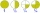The expression 4/12 can be expressed in its lowest term as 1/3. What is 3/15 expressed in its lowest term?
• StudentsThere are 1100 students at a school. 540 students are girls, the rest are boys. 1/10 of girls are left handed. 1/8 of the boys are left handed. Work out the number of left handed students in the school.
• Report 2A School reports students to teacher ratio of 6:1. If there are 45 teachers in the School, how many students are there?
• Simplify 9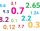Simplify and express the result as a rational number in its simplest form 1/2+ 1/5+ 6.25+0.25
• NumberI think the number. If I add to its third seven I get same as when to its quarter add 8. Which is the number?
• A marketA market vendor was able to sell all the mangoes, papayas, and star apples.  1/5  of the fruits were mangoes,   2/3  of them were papayas and the rest were star apples.  How many parts of the fruits sold are star apples?
• Blue silk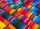There are 4 rolls of blue silk. Each roll holds 6 3/4 yd of silk. It takes 3/5 of a yard to make one necktie. How many necktie can be made from the 4 rolls of blue silk?
• Golf ballsOf the 28 golf balls, 1/7 are yellow. How many golf balls are yellow? Use the model to help you. Enter your answer in the box.
• DIY press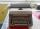Under socialism regime was in some socialist countries to own a typewriter requires special permission. That has hindered the spread of DIY literature (manually transcribed through carbon copy paper for typewriters). Calculate how many typewriters today c
• Chester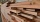Chester has a piece of wood measuring 1 2/3 cm. He needs to cut it to pieces measuring 3/4 cm long. How many pieces of wood did Chester cut?
• Seed germination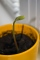Seed germination is 80%. 8% of the germinated plants subsequently die. How many seeds does a gardener have to sow in order to grow 2,000 plants?Skilled workshop master washes client car 1/5 hour, cleaned the client's car in 5/4 hour, and painted small defects on car 1 1/3 hour. How long did it take him to do all the necessary work tasks?